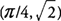## First Derivative Test for Local Extrema

If the derivative of a function changes sign around a critical point, the function is said to have a local (relative) extremum at that point. If the derivative changes from positive (increasing function) to negative (decreasing function), the function has a local (relative) maximum at the critical point. If, however, the derivative changes from negative (decreasing function) to positive (increasing function), the function has a local (relative) minimum at the critical point. When this technique is used to determine local maximum or minimum function values, it is called the First Derivative Test for Local Extrema. Note that there is no guarantee that the derivative will change signs, and therefore, it is essential to test each interval around a critical point.

Example 1: If f(x) = x 4 − 8 x 2, determine all local extrema for the function.

f(x) has critical points at x = −2, 0, 2. Because f'(x) changes from negative to positive around −2 and 2, f has a local minimum at (−2,−16) and (2,−16). Also, f'(x) changes from positive to negative around 0, and hence, f has a local maximum at (0,0).

Example 2: If f(x) = sin x + cos x on [0, 2π], determine all local extrema for the function.

f(x) has critical points at x = π/4 and 5π/4. Because f′(x) changes from positive to negative around π/4, f has a local maximum at. Also f′(x) changes from negative to positive around 5π/4, and hence, f has a local minimum at